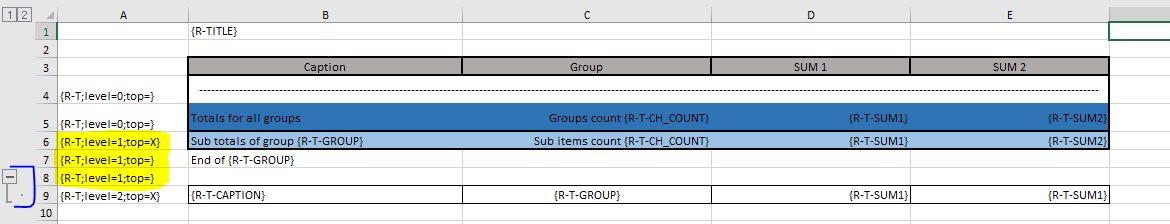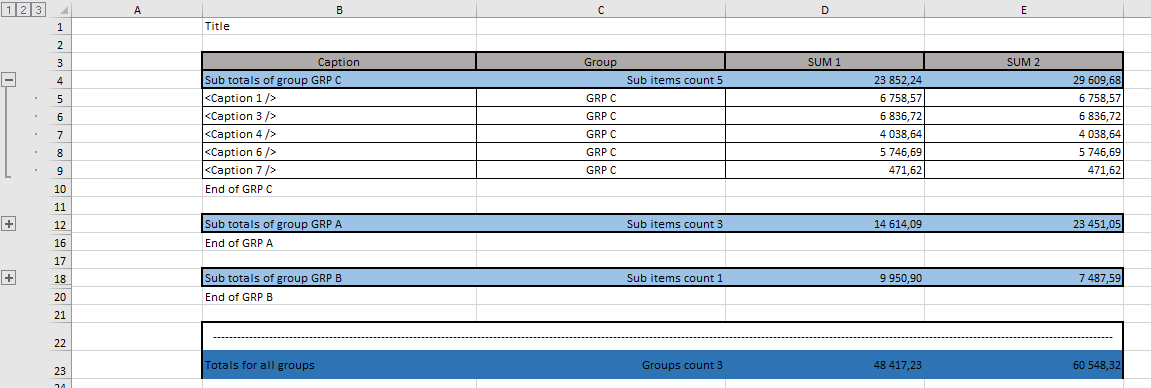If you have ordinary table with simple totals you should use abap internal tables.
But if you also have to display subtotals and other hierarchical structures it’s better to use `trees`.
There are 2 methods to create trees. In this page I’ll try to explain the first one `TREE_CREATE`.
About the `TREE_CREATE_RELAT` you can read in the follwing page.

### TREE_CREATE method

``````    " Document structure
BEGIN OF ts_root,
title TYPE string,
t     TYPE  REF TO data, " better to use general type than zcl_xtt_replace_block=>ts_tree
END OF ts_root.

ls_root-t = zcl_xtt_replace_block=>tree_create(
it_table      = lr_table       " from 7.5 REF #(lt_rows)
iv_fields     = 'GROUP'   ).   " Name of the fields delimited by ;
``````

The simplest way to describe the method is to imagine ALV grid with different subtotals.

`````` iv_fields     = 'BUKRS'        " Subtotals by 1 field
iv_fields     = 'BUKRS;WERKS'  " Subtotals bt 2 fields
``````

to calculate sums (and averages, for example) you should define handler to process tree and subitems

``````      on_prepare_tree_05 FOR EVENT prepare_tree OF zcl_xtt_replace_block
IMPORTING
ir_tree      " Type Ref To ZCL_XTT_REPLACE_BLOCK=>TS_TREE
ir_data      " Type Ref To DATA
ir_sub_data, " Type Ref To DATA
``````

first of all in the handler you have to cast data to original data types

``````  FIELD-SYMBOLS:
<ls_data>     TYPE ts_tree_05,
<ls_sub_data> TYPE ts_tree_05.

" Cast to specific data
ASSIGN:
ir_data->*        TO <ls_data>,
ir_sub_data->*    TO <lt_sub_data>.
``````

and in the loop you can calculate whatever you need

``````  " And calc sums
LOOP AT <lt_sub_data> ASSIGNING <ls_sub_data>.
<ls_data>-sum1  = <ls_data>-sum1 + <ls_sub_data>-sum1.
<ls_data>-sum2  = <ls_data>-sum2 + <ls_sub_data>-sum2.
ENDLOOP.
``````

In the template if you have different rows formatting for each level you can specify them by `;level=` and `;top=` additions. If subitems go after parental item just add `;top=X` for the level, otherwise `;top=` or just omit it.Also if you specify outline level in the template it will be replicated in the final report for the corresponding items.

The result look like in ALV GRID